# How to Calculate and Solve for Electrochemical Cell Potential | Corrosion

The electrochemical cell potential is illustrated by the image below.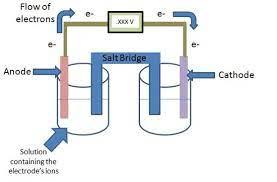To compute for electrochemical cell potential, two essential parameters are needed and these parameters are Positive Cell Potential (V2°) and Negative Cell Potential (V1°).

The formula for calculating electrochemical cell potential:

ΔV° = V2° – V1°

Where:

ΔV° = Electrochemical Cell Potential
V2° = Positive Cell Potential
V1° = Negative Cell Potential

Given an example;
Find the electrochemical cell potential when the positive cell potential is 14 and the negative cell potential is 7.

This implies that;

V2° = Positive Cell Potential = 14
V1° = Negative Cell Potential = 7

ΔV° = V2° – V1°
ΔV° = 14 – 7
ΔV° = 7

Therefore, the elecreochemical cell potential is 7 V.

Calculating the Positive Cell Potential when the Electrochemical Cell Potential and the Negative Cell Potential is Given.

V2° = ΔV° + V1°

Where:

V2° = Positive Cell Potential
ΔV° = Electrochemical Cell Potential
V1° = Negative Cell Potential

Let’s solve an example;
Find the positive cell potential when the electrochemical cell potential is 20 and the negative cell potential is 14.

This implies that;

ΔV° = Electrochemical Cell Potential = 20
V1° = Negative Cell Potential = 14

V2° = ΔV° + V1°
V2° = 20 + 14
V2° = 34

Therefore, the positive cell potential is 34 V.

Calculating the Negative Cell Potential when the Electrochemical Cell Potential and the Positive Cell Potential is Given.

V1° = V2° – ΔV°

Where:

V1° = Negative Cell Potential
ΔV° = Electrochemical Cell Potential
V2° = Positive Cell Potential

Let’s solve an example;
Find the negative cell potential when the electrochemical cell potential is 18 and the positive cell potential is 24.

This implies that;

ΔV° = Electrochemical Cell Potential = 18
V2° = Positive Cell Potential = 24

V1° = V2° – ΔV°
V1° = 24 – 18
V1° = 6

Therefore, the negative cell potential is 6 V.

Nickzom Calculator – The Calculator Encyclopedia is capable of calculating the electrochemical cell potential.

To get the answer and workings of the electrochemical cell potential using the Nickzom Calculator – The Calculator Encyclopedia. First, you need to obtain the app.

You can get this app via any of these means:

You can also try the demo version via https://www.nickzom.org/calculator

Apple (Paid) – https://itunes.apple.com/us/app/nickzom-calculator/id1331162702?mt=8
Once, you have obtained the calculator encyclopedia app, proceed to the Calculator Map, then click on Materials and Metallurgical under Engineering.Now, Click on Corrosion under Materials and MetallurgicalNow, Click on Electrochemical Cell Potential under Corrosion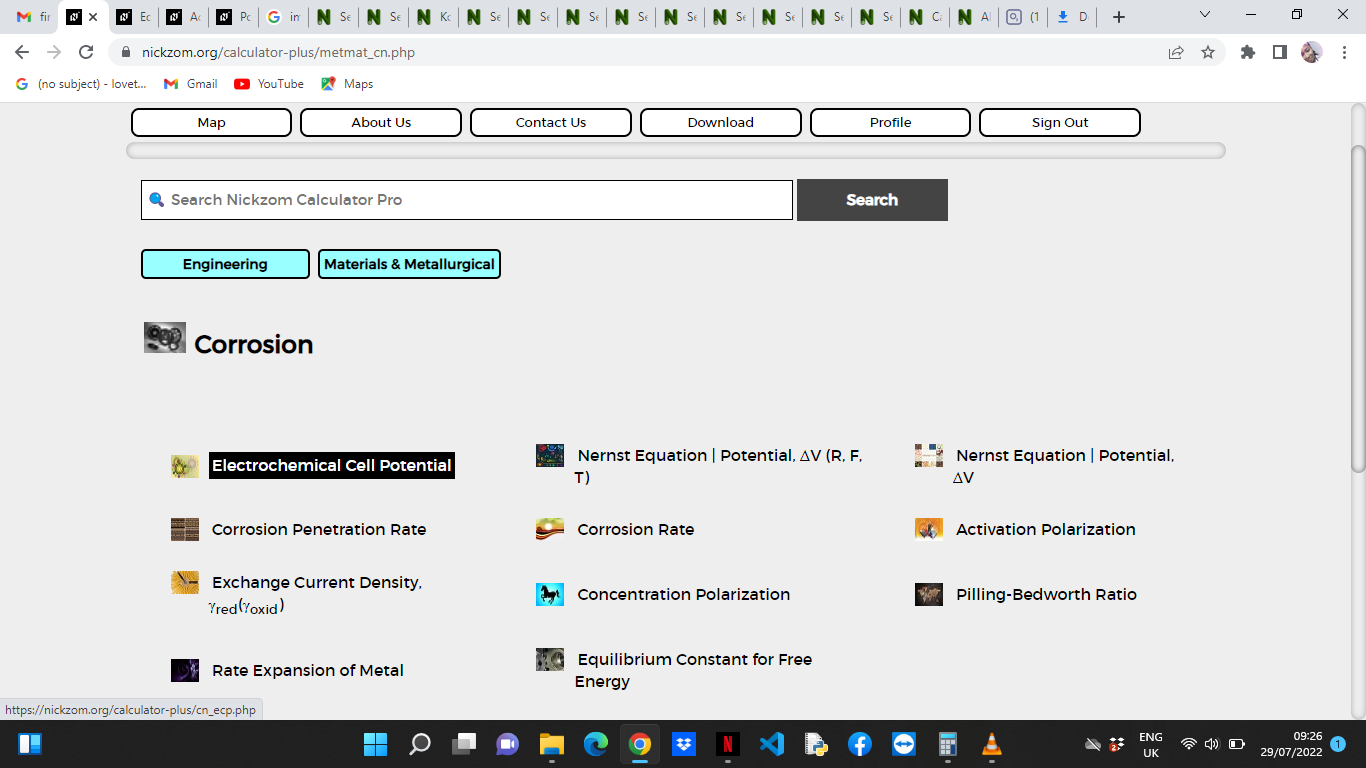The screenshot below displays the page or activity to enter your values, to get the answer for the electrochemical cell potential according to the respective parameter which is the Positive Cell Potential (V2°) and Negative Cell Potential (V1°).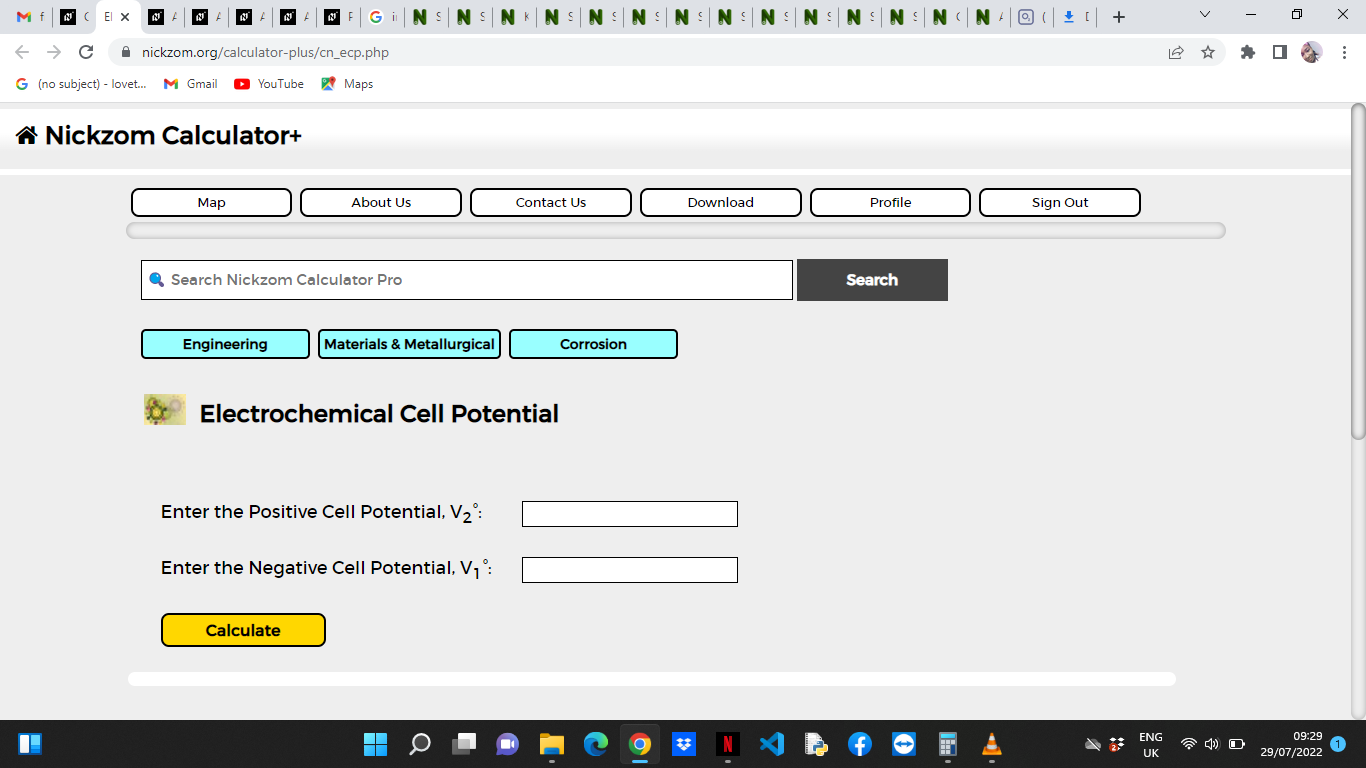Now, enter the values appropriately and accordingly for the parameters as required by the Positive Cell Potential (V2°) is 14 and Negative Cell Potential (V1°) is 7.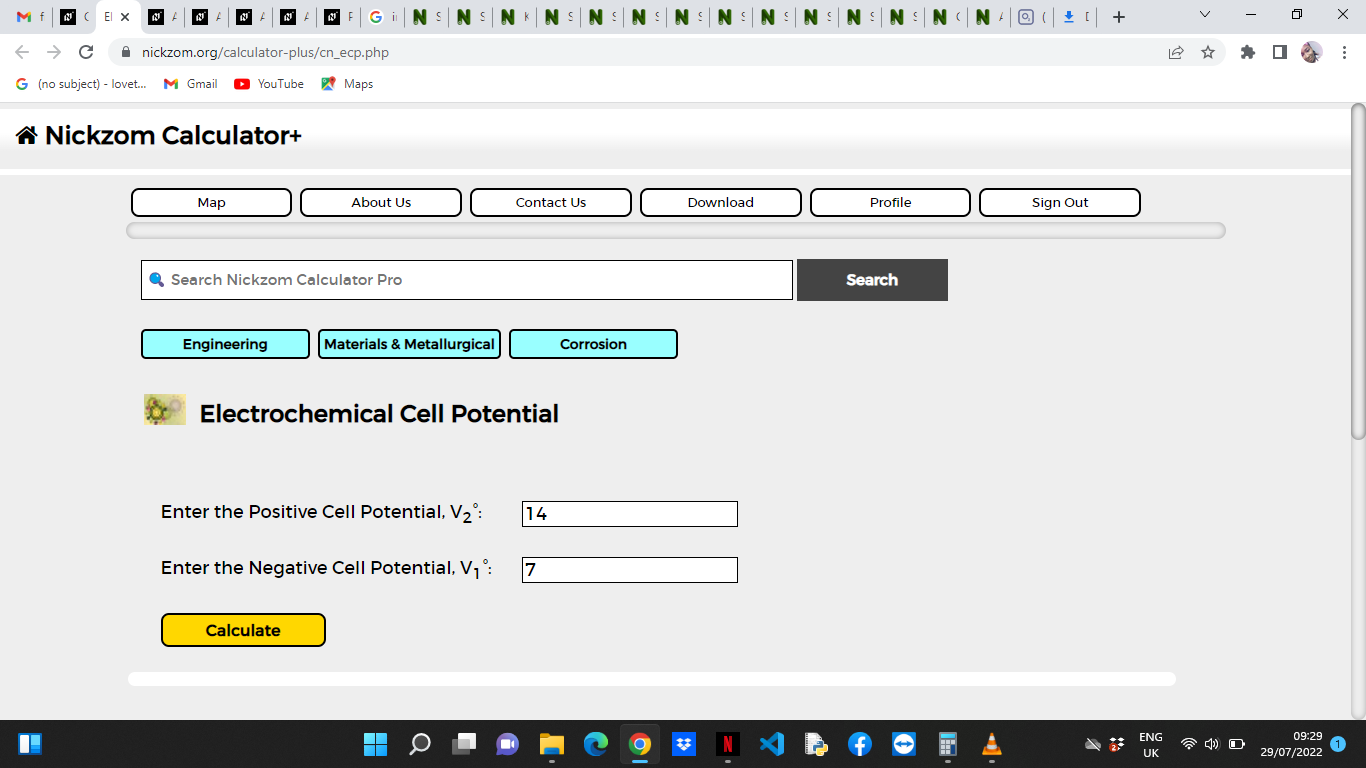Finally, Click on Calculate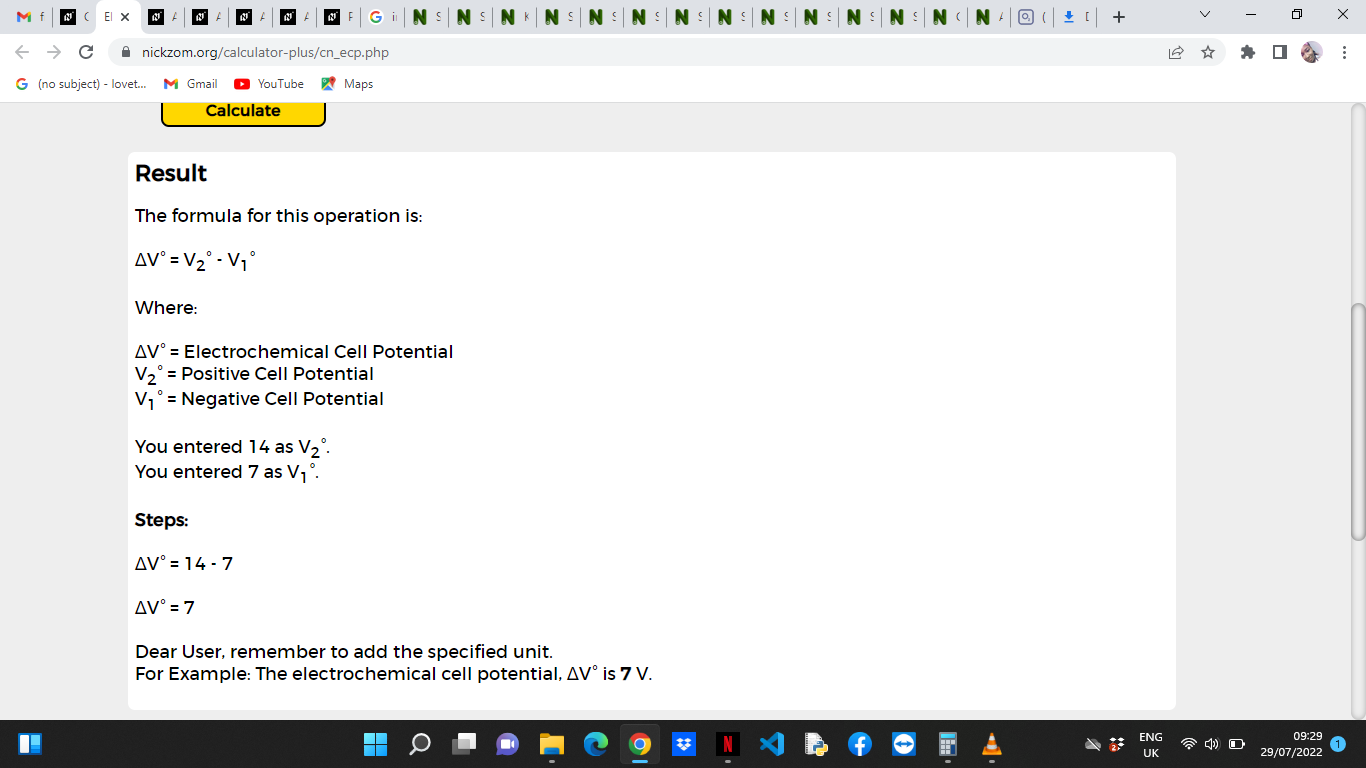As you can see from the screenshot above, Nickzom Calculator– The Calculator Encyclopedia solves for the electrochemical cell potential and presents the formula, workings and steps too.